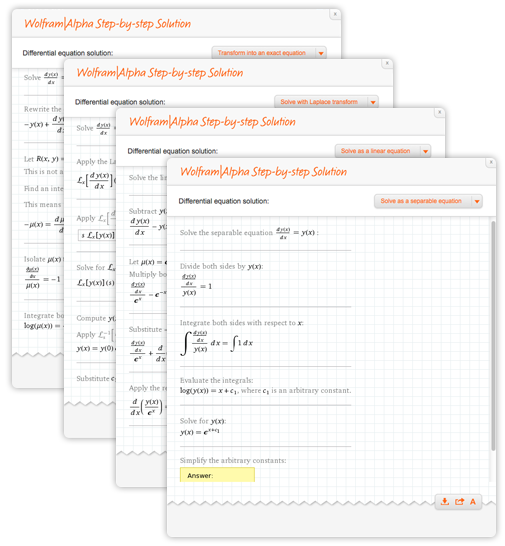# Ordinary Differential Equations (ODEs) Made Easy

February 3, 2014 —Comments Off

As the winter term kicks into gear, you might start hoping you had an ODE-solving pet monkey as the math and physics problem sets start piling up. Now, we do not offer ODE-solving primates at the moment, but we can help you with your differential equations problem sets. Wolfram|Alpha can solve a plethora of ODEs, each using multiple methods.

Let’s look at the simple ODE y‘(x) = y(x). We see Wolfram|Alpha classifies the ODE, solves it, and provides a family of plots. Notice how four methods are provided with the Step-by-step solution.How about we model the position of a spring with resting initial position and velocity, and forcing function sin(2t): y”(t) + y(t) = sin(2t), y(0) = 0, y‘(0) = 0. Three methods are provided here for solving this ODE.An example of an ODE that models the angle of a pendulum over time is y“(t) + sin(y(t)) = 0. The solution for this ODE is in terms of special functions, which is not a problem for Wolfram|Alpha. For small angles, we can approximate this ODE by y”(t) + y(t) = 0.The coverage of differential equations Wolfram|Alpha provides goes much deeper.

Given a function, we can calculate an ODE such that the given function is a solution:

Solve a higher-order differential equation:

Solve a vector ODE:

Solve an ODE with differential notation:

Find the solution to a general class of differential equations:

Solve a PDE:

Calculate solutions to an ODE that are implicit or in terms of special functions:

Interactivity is available for Wolfram|Alpha Pro users. Check out this complex-plane solution curve for free:Wolfram|Alpha can do more with differential equations, such as wronskian of cos(t)+1, sin(t), laplace transform of t^2*sin(t), 1D harmonic oscillator Schrödinger equation, free particle in 2D, throwing with quadratic drag, or forward euler method y‘(x) + y(x) = x, y(0) = 1, h = 0.01.So now you don’t have to choose between violating the dorm’s pet policy and getting your problem set done in a timely fashion. Use Wolfram|Alpha to not only solve your differential equations, but to receive help understanding each step of the solution so that you are better prepared for exams!

Boa tarde.

Instalei o wolfram alpha no meu telemovel mas nao consigo ver as resoluçoes dos problemas.
Por exemplo, quando calculo uma equaçao diferenciavel aparece o resulado, aparece o step by solution mas quando carrego nao aparece a resolução. Sera que me poderia ajudar?

Posted by Débora Ferreira September 1, 2014 at 10:56 am

can you please tell me the mathematical code of inverse sumudu transformation?

Posted by Uroosa Arshad October 22, 2014 at 12:47 am

Thank you for your comment. Unfortunately neither Mathematica nor Wolfram|Alpha support sumudu transformations.

Posted by The Wolfram Team October 22, 2014 at 1:55 pm

I am traying to resolver a differential equation and specify what to resolve,

This is the equation dy/dx=(y+3)/(x-4) I have developed the solution manually and I get as a result y=-(3x/4) and I get another result here at the website. ( I am solving “y” variable)

Can you help me or explaind me what I am doing wrong?

Thanks

Posted by Victor February 12, 2015 at 12:06 am